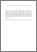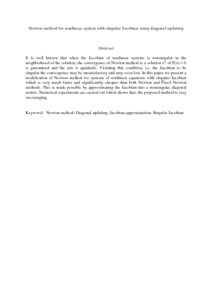# Newton method for nonlinear system with singular Jacobian using diagonal updating

## Citation

Yusuf, Mohammed Waziri and Leong, Wah June and Abu Hassan, Malik and Monsi, Mansor (2010) Newton method for nonlinear system with singular Jacobian using diagonal updating. International Journal of Mathematics and Computations, 8 (S10). pp. 1-8. ISSN 0974-5718; ESSN: 0974-570X

## Abstract

It is well known that when the Jacobian of nonlinear systems is nonsingular in the neighborhood of the solution, the convergence of Newton method to a solution x* of F(x) = 0 is guaranteed and the rate is quadratic. Violating this condition, i.e. the Jacobian to be singular the convergence may be unsatisfactory and may even lost. In this paper we present a modification of Newton method for systems of nonlinear equations with singular Jacobian which is very much faster and significantly cheaper than both Newton and Fixed Newton methods. This is made possible by approximating the Jacobian into a nonsingular diagonal matrix. Numerical experiments are carried out which shows that, the proposed method is very encouraging.Preview
PDF (Abstract)
Newton method for nonlinear system with singular Jacobian using diagonal updating.pdfView Item